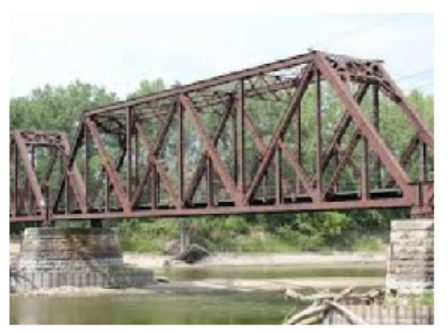# Trigonometry

## Chapter1Triangles and Circles

• $$\displaystyle \blert{\textbf{Angles and Triangles}}$$
• $$\displaystyle \blert{\textbf{Similar Triangles}}$$
• $$\displaystyle \blert{\textbf{Circles}}$$Circles and triangles are the simplest geometric figures. A circle is the simplest sort of curve, and a triangle is the simplest polygon -- the one with the fewest sides. Each has properties that makes it useful in many fields of endeavor. A triangle is the most stable of polygons, because once its sides are fixed in length, its angles cannot change. A triangular truss bridge is a stable structure. A three-legged stool will not wobble.A circle encloses more area then any other figure of the same length, or perimeter, and a sphere encloses more space. A geodesic dome is a portion of a sphere constructed with triangles. It has been called "the strongest, lightest and most efficient means of enclosing space known to man.”
Geodesic domes may also help us learn how to live on another planet. In 2017, the United Arab Emirates began construction on Mars Science City, a series of interconnecting geodesic domes designed to be a realistic model for living on Mars. The city will cover 1.9 million square feet, and its walls will be 3D-printed using sand from the desert.The city will contain laboratories to address food, sustainability, and energy issues all over the world. Finally, the project will implement an experiment wherein a team will spend a year living in the simulated planet for a year.## Activity1.1.Properties of Triangles.

Materials: You will need paper and pencil, scissors, a ruler and compass, and two plastic straws.
Prepare: Cut the straw into pieces of lengths 2 inches, 3 inches, and 6 inches. Use the ruler to draw a large triangle, and cut it out.
1. What do we know about the sides of a triangle?
1. Can you make a triangle with sides of length 2 inches, 3 inches, and 6 inches?
2. Use the pieces of length 2 inches and 3 inches to form two sides of a triangle. What happens to the length of the third side as you increase the angle between the first two sides?
3. What is the longest that the third side could be? What is the smallest?
1. Two sides of a triangle are 6 centimeters and 8 centimeters long. What are the possible lengths of the third side?
2. Two sides of a triangle are $$p$$ units and $$q$$ units long. What are the possible lengths of the third side?
2. What do we know about the angles of a triangle?
1. Use a protractor to measure the three angles of the paper triangle in degrees. Now add up the three angles. What is their sum?
2. Tear off the three corners of the triangle. Place them side-by-side with their vertices (tips) at the same point. What do you find?
3. How are the sides and angles of a triangle related?
1. A standard way to label a triangle is to call the angles $$A,~B,$$ and $$C\text{.}$$ The side opposite angle $$A$$ is called $$a\text{,}$$ the side opposite angle $$B$$ is called $$b\text{,}$$ and the side opposite angle $$C$$ is called $$c\text{.}$$ Sketch a triangle and label it with standard notation.
2. Using a ruler, carefully draw a triangle and label it with standard notation so that $$a \gt b \gt c\text{.}$$ Now use a protractor to measure the angles and list them in order from largest to smallest. What do you observe?
3. Using a ruler, carefully draw another triangle and label it with standard notation so that $$A \gt B \gt C\text{.}$$ Now use a ruler to measure the sides and list them in order from largest to smallest. What do you observe?
4. What do we know about right triangles?
1. The side opposite the $$90\degree$$ angle in a right triangle is called the hypotenuse. Why is the hypotenuse always the longest side of a right triangle?
2. The Pythagorean theorem states that:
 IF: $$a\text{,}$$ $$b\text{,}$$ and $$c$$ are the sides of a right triangle, and $$c$$ is the hypotenuse THEN: $$\qquad$$ $$a^2+b^2=c^2$$
The "if" part of the theorem is called the hypothesis, and the "then" part is called the conclusion. The converse of a theorem is the new statement you obtain when you interchange the hypothesis and the conclusion. Write the converse of the Pythagorean theorem.
3. The converse of the Pythagorean theorem is also true. Use the converse to decide whether each of the following triangles is a right triangle. Support your conclusions with calculations.
1. $$a = 9\text{,}$$ $$~ b = 16\text{,}$$ $$~ c = 25$$
2. $$a = 12\text{,}$$ $$~ b = 16\text{,}$$ $$~ c = 20$$
3. $$a = \sqrt{8}\text{,}$$ $$~ b = \sqrt{5}\text{,}$$ $$~ c = \sqrt{13}$$
4. $$a = \dfrac{\sqrt{3}}{2}\text{,}$$ $$~ b = \dfrac{1}{2}\text{,}$$ $$~ c = 1$$